# RS Aggarwal Class 7 Solutions Chapter 11 Profit and Loss Ex 11A

In this chapter, we provide RS Aggarwal Solutions for Class 7 Chapter 11 Profit and Loss Ex 11A for English medium students, Which will very helpful for every student in their exams. Students can download the latest RS Aggarwal Solutions for Class 7 Chapter 11 Profit and Loss Ex 11A Maths pdf, free RS Aggarwal Solutions Class 7 Chapter 11 Profit and Loss Ex 11A Maths book pdf download. Now you will get step by step solution to each question.

### RS Aggarwal Solutions for Class 7 Chapter 11 Profit and Loss Ex 11A Download PDF

Question 1.
Solution:
(i) C.P. = Rs. 950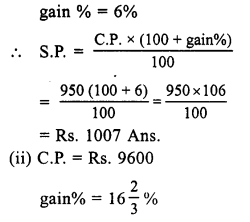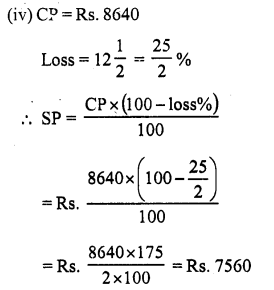Question 2.
Solution:
(i) CP = Rs. 2400
SP = Rs. 2592
Gain = SP – CP = Rs. 2592 – Rs. 2400 = Rs. 192Question 3.
Solution:
(i) S.P. = Rs. 924
Gain% = 10%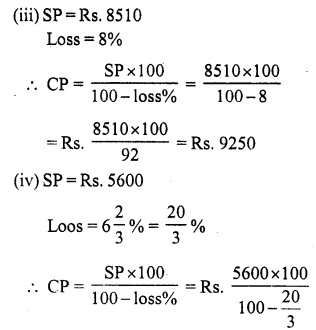Question 4.
Solution:
C.P. of an almirah = Rs. 13600
Amount spent on transportation = Rs. 400
Total cost price (CP) = Rs. 13600 + Rs. 400 = Rs. 14000
SP = Rs. 16800
Total gain = SP – CP
= Rs. 16800 – Rs. 14000 = Rs. 2800Question 5.
Solution:
CP of an old house = Rs. 765000
Amount spent on repairs = Rs. 115000
Total cost price (CP) = Rs. 765000 + Rs. 115000 = Rs. 880000
Gain = 5%Question 6.
Solution:
CP of 1 dozen or 12 lemons = Rs. 25
SP of 5 lemons = Rs. 12Question 7.
Solution:
SP of 12 pens = CP of 15 pens
Let CP of 1 pen = Re. 1
The CP of 15 pens = Rs. 15
and SP of 12 pens = Rs. 12Question 8.
Solution:
SP of 16 spoons = CP of 15 spoons
Let CP of 1 spoon = Re. 1
Then CP of 15 spoons = Rs. 15
and SP of 16 spoons = Rs. 15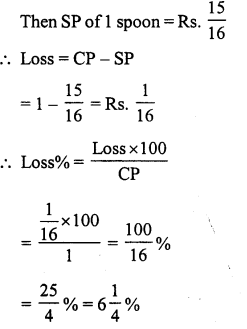Question 9.
Solution:
For Manoj
CP of video = Rs. 12000
Gain = 10%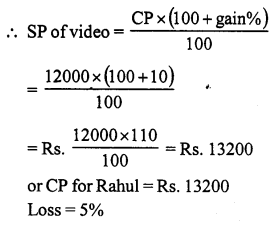Question 10.
Solution:
SP of sofa set = Rs. 21600
Gain = 8%Question 11.
Solution:
SP of a watch = Rs. 11400
Loss = 5%Question 12.
Solution:
SP of calculator = Rs. 1325
Gain = 6%Question 13.
Solution:
SP of computer = Rs. 24480
Loss = 4%Question 14.
Solution:
In first case gain =15%
and in second case, gain = 20%
Difference = 20 – 15 = 5%
Now 5% of the CP = Rs. 108
CP = 108×1005 = Rs. 2160

Question 15.
Solution:
In first case, loss = 8%
and in second case gain = 6%
Difference = 8 + 6 = 14%
Now 14% of CP = Rs. 3360
CP = Rs. 3360×10014 = Rs. 24000

Question 16.
Solution:
SP of first cycle = Rs. 2376
Gain = 10%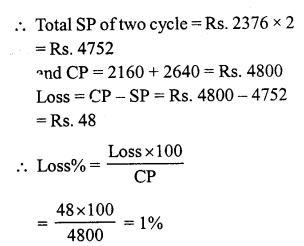Question 17.
Solution:
SP of an exhaust fan = Rs. 7350Question 18.
Solution:
Let CP of watch for Mohit = Rs. 100
Gain =10%
SP for Mohit = Rs. 100 + 10 = Rs. 110
CP for Karim = Rs. 110
Gain = 4%Question 19.
Solution:
Retail price or S.P. of the machine for retailer = Rs. 37950
Gain = 25%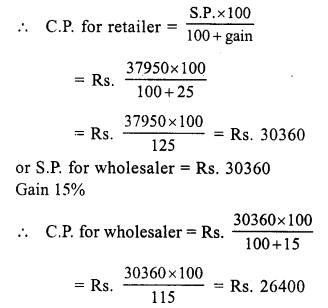Question 20.
Solution:
CP of video = Rs. 20000
and CP of television = Rs. 30000
Loss on video = 5%
and gain on TV = 8%Total CP of video and TV = Rs. 20000 + 30000 = Rs. 50000
and SP of video and TV = Rs. 190000 + 32400 = RS. 51400
Gain = SP – CP = Rs. 51400 – 50000 = Rs. 1400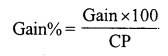Question 21.
Solution:
SP of 36 oranges = CP of 36 oranges – loss = CP of 36 oranges – SP of 4 oranges
⇒ SP of 36 oranges + SP of 4 oranges = CP of 3 6 oranges
⇒ SP of 40 oranges = CP of 36 oranges
Let CP of each orange = Re. 1
CP of 36 oranges = Rs. 36
and SP of 40 of 40 oranges = Rs. 36Question 22.
Solution:
We know that C.P. = S.P. – gain
C.P. of 8 dozen pencils = S.P. of 8 dozen pencils – S.P. of 1 dozen pencils
= S.P. of 7 dozen pencils = Rs. 100 (suppose)
C.P. of 1 dozen = Rs.1008All Chapter RS Aggarwal Solutions For Class 7 Maths

—————————————————————————–

All Subject NCERT Exemplar Problems Solutions For Class 7

All Subject NCERT Solutions For Class 7

*************************************************

I think you got complete solutions for this chapter. If You have any queries regarding this chapter, please comment on the below section our subject teacher will answer you. We tried our best to give complete solutions so you got good marks in your exam.

If these solutions have helped you, you can also share rsaggarwalsolutions.in to your friends.